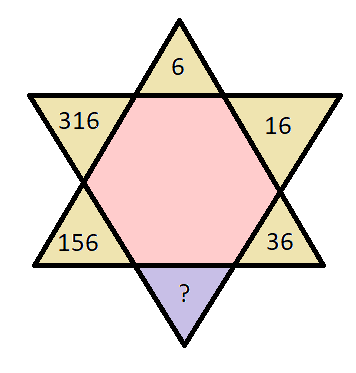# QOTD- The numbers in the figure given below follows a certain pattern. Study the pattern and chose the number which will replace the question mark?

2019-07-31 | Team PendulumEduOptions:

A. 78

B. 76

C. 72

D. 74

Solution:

Considering the given numbers in the star in a clockwise manner starting from ‘16’ we get,

16 = 6 * 2 + 4

36 = 16 * 2 + 4

(?) = 36 * 2 + 4 = 76

156 = 76 * 2 + 4

316 = 156 * 2 + 4

Hence, B is the required solution.

Non-verbal series questions are asked in various government exams like SSC CGL, SSC CHSL, SSC MTS, SSC CPO, RRB JE, RRB NTPC, RRB GROUP D, etc. Try and attempt free mock tests at PendulumEdu and practice exam oriented logical reasoning questions. PendulumEdu also offers one-to-one session for clearing all types of doubts related to various exams.0